Courses

# HC Verma Test: Electrostatic Potential & Capacitance

## 45 Questions MCQ Test Physics For JEE | HC Verma Test: Electrostatic Potential & Capacitance

Description
This mock test of HC Verma Test: Electrostatic Potential & Capacitance for Class 12 helps you for every Class 12 entrance exam. This contains 45 Multiple Choice Questions for Class 12 HC Verma Test: Electrostatic Potential & Capacitance (mcq) to study with solutions a complete question bank. The solved questions answers in this HC Verma Test: Electrostatic Potential & Capacitance quiz give you a good mix of easy questions and tough questions. Class 12 students definitely take this HC Verma Test: Electrostatic Potential & Capacitance exercise for a better result in the exam. You can find other HC Verma Test: Electrostatic Potential & Capacitance extra questions, long questions & short questions for Class 12 on EduRev as well by searching above.
*Multiple options can be correct
QUESTION: 1

Solution:
QUESTION: 2

### The capacitance of a metallic sphere will be 1mF, if its radius is nearly

Solution:

The expression of capacitance of metallic sphere is C=4πϵ0​r (The capacitance of a single spherical conductor means that we imagine a spherical shell of infinite radius surrounding the first conductor.)
Therefore,

r = C/4πϵ​0

= 10 − 6 × 9 × 109 = 9 × 103 m = 9km

*Multiple options can be correct
QUESTION: 3

### Two capacitors of capacitances 1mF and 3mF are charged to the same voltages 5V. They are connected in parallel with oppositely charged plates connected together. Then

Solution:
QUESTION: 4

Two spherical conductors A and B of radii R and 2R respectively are each given a charge Q. When they are connected by a metallic wire. The charge will

Solution:
QUESTION: 5

The capacity of a parallel plate condenser is C. Its capacity when the separation between the plates is halved will be

Solution:

The capacity of a parallel plate condenser is given as,
C=εA/​d
When the separation between the plates is halved then the new capacity of the condenser is given as,
C′=εA​/(d/2)​
C′=2C
Thus, the new capacity of parallel plate condenser is 2C.

QUESTION: 6

If the p.d. across the ends of a capacitor 4mF is 1.0 kilovolt. Then its electrical potential energy will be

Solution: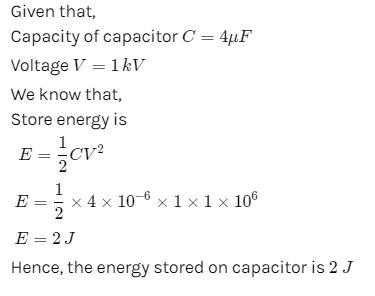QUESTION: 7

The energy of a charged capacitor resides in

Solution:

The energy of a charged capacitor resides in both electric and magnetic fields .
Energy resides in the electric field because of the charges on the capacitor.
Energy resides in the magnetic field because of The Maxwell's displacement current in the capacitor.

QUESTION: 8

The capacitance of a parallel plate condenser does not depend upon

Solution:

The capacitance C is the amount of charge stored per volt, or C=QV. The capacitance of a parallel plate capacitor is C=ϵ0Ad, when the plates are separated by air or free space.

QUESTION: 9

The energy density in a parallel plate capacitor is given as 2.2 × 10-10 J/m3. The value of the electric field in the region between the plates is -

Solution: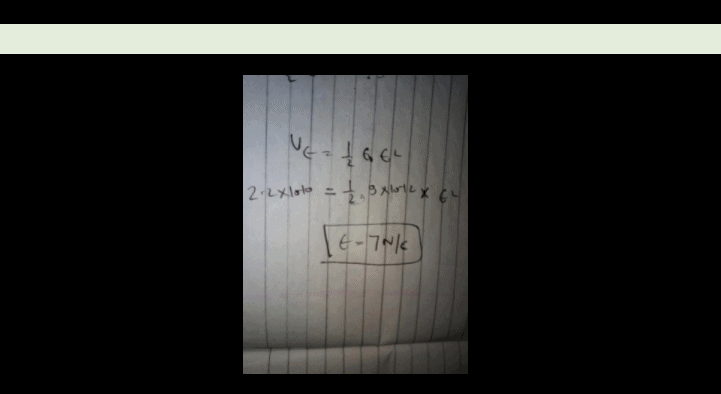QUESTION: 10

Two capacitances of capacity C1 and C2 are connected in series and potential difference V is applied across it. Then the potential difference acros C1 will be

Solution: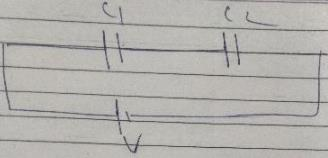QUESTION: 11

A conductor of capacitance 0.5mF has been charged to 100volts. It is now connected to uncharged conductor of capacitance 0.2mF. The loss in potential energy is nearly

Solution:

Given,
C1=0.5 mf
C2=0.2mf
V=100v
So,
U=(1/2) CTV2
CT=C1C2/XC1+C2=(0.5x0.2/0.5+0.2)mf
CT=0.14mf
U=(1/2)(0.14mf)(100V)2
=(10.07x1-6x104)J
=(7x10-2x10-6x104)J
=7x10-4J

QUESTION: 12

Two spherical conductors of capacitance 3.0mF and 5.0mF are charged to potentials of 300volt and 500volt. The two are connected resulting in redistribution of charges. Then the final potential is

Solution:

First find the charge in 1st capacitor
Q1= C1xV1
=0.003*300
=0.9 C
Similarly, find Q2
i.e. Q2=2.5 C
After connecting the capacitors in parallel you get a net capacitance C=8 mF (c1+c2).
Thus, final potential is V=Q/C
Q=Q1+Q2=3.4 C
V=3.4/0.008
= 425 V

QUESTION: 13

A parallel plate capacitor of capacitance C is connected to a battery and is charged to a potential difference V. Another capacitor of capacitance 2C is similarly charged to a potential difference 2V. The charging battery is now disconnected and the capacitors are connect in parallel to each other in such a way that the positive terminal of one is connected to the negative terminal of the other. The final energy of the configuration is

Solution:

Where N is the number of turns of the toroid coil, I is the amount of current flowing and r is the radius of the toroid.
As both capacitors are in parallel so equivalent capacitance Ceq​=C+2C=3C .
Net potential, Vn​=2V−V=V
Energy stored, U=(1/2)​Ceq​Vn2​=(1/2)×3CV2=(3/2)​CV2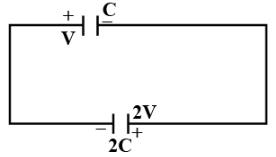QUESTION: 14

A 2μF capacitor is charged to a potential = 10 V. Another 4μF capacitor is charged to a potential = 20V. The two capacitors are then connected in a single loop, with the positive plate of one connected with negative plate of the other. What heat is evolved in the circuit?

Solution:

Charge on 2μf capacitor=10vx2μF=20μC=Q1
Charge on 4μf capacitor=4μFx20v=80μC=Q2
Now, these capacitors are connected in a single loop in given manner,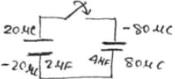Now after transfer,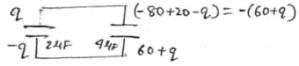Applying loop rule,
(q/2)+[(60+q)/4]=0=>q=-20μC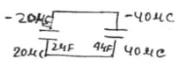Now, drop across the capacitors is,
ΔVf=40/4v=10v
Final energy stored in capacitor=Uf
= (1/2) (C1+C2)(ΔVf)2=1/2 x6 x100uf=300 μf
Initial energy stored in capacitor=Ui
= (1/2) C1V12+(1/2) C2V22=(1/2)x2x100+ (1/2)x4x400=900 μf
Now, heat evolved in the circuit will be,
Ui-Uf= (900-300) μf=600 μf
Thus, the correct answer would be option B.

*Multiple options can be correct
QUESTION: 15

The figure shows a diagonal symmetric arrangement of capacitors and a battery
Identify the correct statements.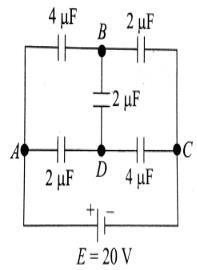Solution:

If 2μF where not present between B and D, then potential drop across upper 4μF will be less than potential drop across lower 2μF,i.e.,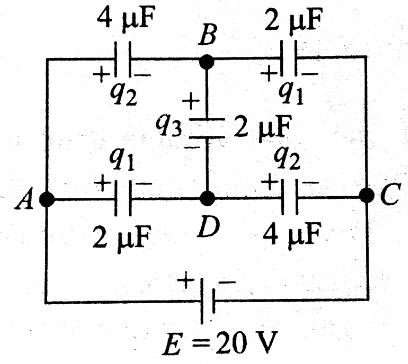QUESTION: 16

In the circuit shown in figure charge stored in the capacitor of capacity 5μf is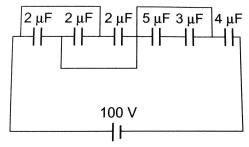Solution:
QUESTION: 17

Plate A of a parallel air filled capacitor is connected to a spring having force constant k and plate B is fixed. If a charge +q is placed on plate A and charge _q on plate B then find out extension in spring in equilibrim. Assume area of plate is `A'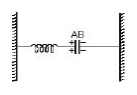Solution:At equilibrium,
Felectric=Fspring
qE=kx ____(1)
we know that,
C=Q/V
And V=ε.d
C=Aε0/d
Therefore, ε=θ/Aε0
Here, ε=q/ Aε0
Putting the value in the equation (1)
q/ Aε0=kx
x=q2/akε0

QUESTION: 18

Three uncharged capacitors of capacitane C1 = 1mF, C2 = 2mF and C3 = 3mF are connected as shown in figure to one another and to points A, B and D potential fA = 10V, fB = 25V and fD = 20 V, Determine the potential (f0) at point O.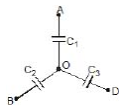Solution:

⇒q1​+q2​+q3​=0
⇒C1​(Vo​−VA​)+C2​(Vo​−VB​)+C3​(Vo​−VD​)=0
⇒(C1​+C2​+C3​)Vo​=C1​VA​+C2​VB​+C3​VD
Vo​= C1​VA​+C2​VB​+C3​VD​​/ C1​+C2​+C3
= [(1×10)+(2×25)+(3×20)​]/(1+2+3)
=(10+50+60)/6
​=20Volts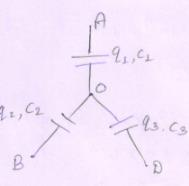QUESTION: 19

Five capacitors are connected as shown in the figure. Initially S is opened and all capacitors are uncharged. When S is closed, steady state is obtained. Then find out potential difference between the points M and N.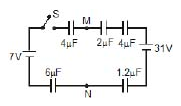Solution:

Five capacitors 4μF, 2μF, 4μF, 6μF and 1.2μF are connected as shown in the figure.
Here, Equivalent capacitance = ½,
EMF = 24
Thus, charge passes through each capacitor = 12 μC
Voltage across 4μF = 3V
Voltage across 6μF = 2V
=> Potential difference (V):
V = 3 + 7+ 2 = 12V
Thus, potential difference (V) between the two given points M and N is 12v.

QUESTION: 20

Find the potential difference Va - Vb between the points a and b shows in each parts of the figure.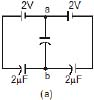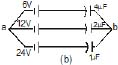Solution:

a.In this figure, the left and the right branch is symmetry. So the current go to the branch 'ab' for both sides are opposite and equal. Hence it cancels out.
Thus, net charge, Q= 0. ∴V=Q/C​=0/C​=0
∴Vab​=0
b.The net potential, V= Net charge /Net capacitance ​=C1​V1​+C2​V2​+C3​V3/7​​
V=(24+24+24​)/7=72/7​=10.28V

QUESTION: 21

Each plate of a parallel plate air capacitor has an area S. What amount of work has to be performed to slowly increase the distance between the plates from x1 to x2 If the charge of the capacitor, which is equal to q or

Solution:

Given,
Area of plate-S
Initial distance=X1
Final distance=X2
Charge given, q
Initial capacitance
C1=Sε/ X1
Energy stored
V1=q2/2C=q2/2(Sε/X1)
U1= q2X1/2Sε
Final capacitance
C2= Sε/X2
Energy stored,
U2= q2 x2/2Sε
Amount of work done=Change in stored energy between capacitor
=Uf-Ui=U2-U1
=(q2X2/2Sε)- (q2X1/2Sε)
Work done=q2/2Sε (X2-X1)

QUESTION: 22

Each plate of a parallel plate air capacitor has an area S. What amount of work has to be performed to slowly increase the distance between the plates from x1 to x2 If the voltage across the capacitor, which is equal to V, is kept constant in the process.

Solution:

When voltage is kept const., the force acing on each plate of capacitor will depend on the distance between the plates.
From energy conversion,
Uf-Ui=Acell+Aagent
Or,(1/2)(ε0S/x2)V2-(1/2)(ε0S/x1)V2
=[(ε0S/x2)- (ε0S/x1)] V2 +Aagent
(as Acell=(qf-q1)V=(Cf-Ci)V2)
So, Aagent= (ε0SV2/2)[(1/x1)-)1/x2)]

QUESTION: 23

If charge on left plane of the 5F capacitor in the circuit segment shown in the figure is-20C, the charge on the right plate of 3F capacitor is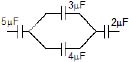Solution:

If the charge on left plate of 5μF is −20μC, then the charge on right plate of 5μF is +20μC
So due to the polarization the charge on the left plate of 3μF is negative and the charge on the right plate of 3μF is positive.
thus,  charge on right plate of 3μF is Q=[3/(3+4)]×20=60/7​=8.57μC

QUESTION: 24

In the circuit shown, the energy stored in 1μF capacitor is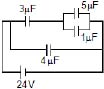Solution:

The capacitance 4 and (3,5,1) are in parallel so potential across (3,5,1) will be V=24V.
The equivalent capacitance of (3,5,1) is Ceq​=3(5+1)/{3+(5+1)}​=2μF
and Qeq​=Ceq​V=2×24=48μC
As 3 and (5,1) are in series so charge on 3 and (5,1) is equal to Qeq​.
Thus potential across (5,1) is V51​= Qeq​​/C51​=48/(5+1)​=8V
As 5 and 1 are in parallel so potential difference of capacitors will be same i.e 8V
Now energy stored in 1 is =(1/2)​C1​V512​=(1/2)​×10−6×82=32μJ

QUESTION: 25

A capacitor C1 = 4mF is connected in series with another capacitor C2 = 1mF. The combination is connected across a d.c. source of voltage 200V. The ratio of potential across C1 and C2 is

Solution:

Voltage in series connections are,
V1=C2/{(C1+C2) x V}  V2={C1/(C1+C2)xV}]
V1=(1/S) x 200
V2=(4/S) x200
Ration, V1/V2=((1/S) x200)/((4/S)x200)=1:4

QUESTION: 26

A 5.0 mF capacitor having a charge of 20 mC is discharged through a wire of resistance of 5.0 W. Find the heat dissipated in the wire between 25 to 50 ms after the capactions are made.

Solution:
QUESTION: 27

A charged capacitor is allowed to discharge through a resistance 2Ω by closing the switch S at the instant t = 0. At time t = ln2μs, the reading of the ammeter falls half of its initial value. The resistance of the ammeter equal to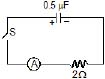Solution:
QUESTION: 28

A capacitor C =100μF is connected to three resistor each of resistance 1 kW and a battery of emf 9V. The switch S has been closed for long time so as to charge the capacitor. When switch S is opened, the capacitor. Discharges with time constant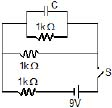Solution:
QUESTION: 29

In the transient shown the time constant of the circuit is :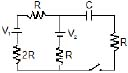Solution:

Applying Thevenin's theorem we consider the circuit from right side and short circuiting the voltage sources and opening the extreme right resistance we get the equivalent resistance
=1/Rth​​=(1/3R)​+(1/R)​=4/3R=3R/4​ Rth​=Thevenin’s resistance
So now adding the remaining resistance R with Rth​ in series we get the equivalent resistance
Req​=(3R/4)​+R=7R/4​
And time constant τ=RC
τ=(7/4)​RC

QUESTION: 30

In the circuit shown in figure C1 =2C2 . Switch S is closed at time t=0. Let i1 and i2 be the currents flowing through C1 and C2 at any time t, then the ratio i1 / i2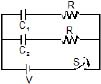Solution:
*Multiple options can be correct
QUESTION: 31

The two plates X and Y of a parallel plate capacitor of capacitance C are given a charge of amount Q each. X is now joined to the positive terminal and Y to the negative terminal of a cell of emf E = Q/C.

Solution:

The answer provided in the forum is wrong. the correct answer is option A.B,C.
Solution:
As the emf of cell is ϵ=Q/C, charge of amount Q will flow from the positive terminal to the negative terminal of the cell through the capacitor.
As the Y is connected to the negative terminal of the cell so it is grounded and the total charge on plate Y will be zero.
Here cell charge the capacitor plate X and its total charge =(Q+Q)=2Q
The energy will supply by cell is U=(½)​Cϵ2
The correct option is A B C only.

*Multiple options can be correct
QUESTION: 32

Two capacitors of capacitances 1mF and 3mF are charged to the same voltages 5V. They are connected in parallel with oppositely charged plates connected together. Then

Solution:

V=(C1V1−C2V2)/C1+C2
=3×5−1×5/1+3
= 2.5volt

*Multiple options can be correct
QUESTION: 33

Each plate of a parallel plate capacitor has a charge q on it. The capacitor is now connected to a battery. Now,

Solution:
*Multiple options can be correct
QUESTION: 34

When a parallel plates capacitor is connected to a source of constant potential difference,

Solution:
*Multiple options can be correct
QUESTION: 35

When two identical capacitors are charged individually to different potentials and connected parallel to each other, after disconnecting them from the source

Solution:
*Multiple options can be correct
QUESTION: 36

Two thin conducting shells of radii R and 3R are shown in the figure. The outer shell carries a charge +Q and the inner shell is neutral. The inner shell is earthed with the help of a switch S.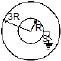Solution:

(A)  Potential of inner shell is due to potential developed by outer shell.
(B)  Since the inner shell is Earthed so its potential becomes zero.
(C)  Vinner=(KQ/3R)+(KQI/R)=0⇒rQ'=−Q/3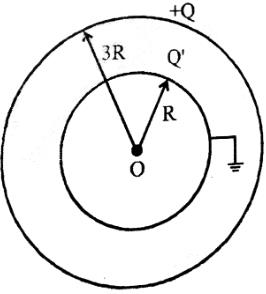(D)  It capacitance becomes =4π∈0R1R2/R2−R1+4π∈R2
Therefore the correct answer is option A B C D.

*Multiple options can be correct
QUESTION: 37

Two capacitors of 2mF and 3mF are charged to 150 volt and 120 volt respectively. The plates of capacitor are connected as shown in the figure. A discharged capacitor of capacity 1.5 mF falls to the free ends of the wire. Then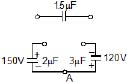Solution:

Charge in 2μF Capacitor =300μC
Charge in 3μF Capacitor =360μC
when 1.5μF falls on the circuit ends, Redistribution of charge now will be:
Charge in 2μF Capacitor =300−xμC
Charge in 3μF Capacitor =360−xμC

Charge in 1.5μF Capacitor =xμC
By Kirchoff Voltage Law:
x/1.5=[(300−x)/2]​+[(360−x)/3]​
which gives x=180μC
Therefore, Answers are A, B & C

*Multiple options can be correct
QUESTION: 38

In the circuit shown initially C1, C2 are uncharged. After closing the switch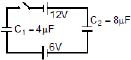Solution:

Here C1​ and C2​ are in series and the total potential between the capacitors is VT​=12+6=18V
Ceq​=(4×8​)/(4+8)=(8/3) ​μF and
Qeq​=Ceq​VT​=(8/3)​×18=48μC
As they are in series so the charge on each capacitor is equal to Qeq​=48μC.
∴Q1​=Q2​=48μC
Potential across C1​ is V1​= Q1/C1​ ​​=48/4​=12V
Potential across C2​ is V2​= Q2​​/C2=48/8​=6V

*Multiple options can be correct
QUESTION: 39

A circuit shown in the figure consists of a battery of emf 10V and two capacitance C1 and C2 of capacitances 1.0mF and 2.0mF respectively. The potential difference VA _ VB is 5V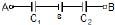Solution:

Here the capacitors are in series so the charge on each is equal to equivalent charge.
The equivalent capacitance is Ceq​=(1×2)/(1+2) ​=(2/3)​μF and the equivalent charge , Qeq​=Ceq​V=(2/3)​(10+5)=10μC
Thus, Q1​=Q2​=Qeq​=10μC
Potential across C1​ is V1​=Q1​/C1​=10/1=10V and Potential across C2​ is V2​=Q2​/C2​=10/2=5V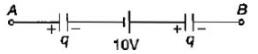*Multiple options can be correct
QUESTION: 40

Two capacitors of equal capacitance (C1 = C2) are shown in the figure. Initially, while the switch S is open, one of the capacitors is uncharged and the other carries charge Q0. The energy stored in the charged capacitor is U0. Sometimes after the switch is colsed, the capacitors C1 and C2 carry charges Q1 and Q2, respectively, the voltages across the capacitors are V1 and V2, and the energies stored in the capacitors are U1 and U2. Which of the following statements is INCORRECT ?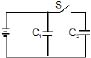Solution:

In the above diagram, C1​ and C2​ are connected in parallel.
Hence, both get the same voltage. ∴V1​=V2​ is correct.
We know that Q1​=C1​V1​ and Q2​=C2​V2
∵C1​=C2​,     Q1​=Q2​
We know that energy U1​=(1/2)​C1​V12​ and U2​=(1/2)​C2​V22
By closing the switch, the C1​ or V1​ doesn't get affected.
Hence U0​=U1​ and not U1​+U2​
Similarly, by closing the switch, the Q1​ or V1​ doesn't get affected.
Hence Q0​=Q1
∵Q0​=Q1​=Q2​,    Q0​=(1/2)​(Q1​+Q2​)

*Multiple options can be correct
QUESTION: 41

The figure shows a diagonal symmetric arrangement of capacitors and a battery

Identify the correct statements.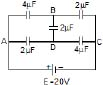Solution:

Now VA−VB<VA−VD⇒VB>VD
Now if 2μF is connected between B and D, charge will flow from B and D and Finally VB>VD.
VA=20V. At junction B,
q2=q1+q3
or (VA−VB)=(VB−VD)2+(VB−VC)2
or 4(VA−VB)+2(VD−VB)=2VB
At junction D:
q2=q1+q3
or 4(VD−VC)=2(VA−VD)+2(VB−VD)
or 2(VA−VD)+2(V,−VD)=4VD.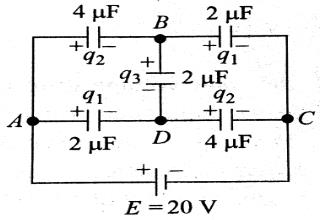*Multiple options can be correct
QUESTION: 42

The figure shows a diagonal symmetric arrangement of capacitors and a batteryIf the potential of C is zero, then

Solution:
QUESTION: 43

For very low frequencies, capacitor acts as _______

Solution:

Capacitive impedance is inversely proportional to frequency. Hence at very low frequencies the impedance is almost infinity and hence acts as an open circuit and no current flows through it.

*Multiple options can be correct
QUESTION: 44

The charge across the capacitor in two different RC circuits 1 and 2 are plotted as shown in figure.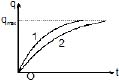Choose the correct statement (s) related to the two circuits

Solution:

The charge on capacitor at any time t is q=qmax​(1−e−t/CR)
As the qmax​ of both curves is the same so both capacitors will be charged to the same charge.
As (2) curves take more time for maximum charging so the time constant of curve (2) is greater than (1). i.e, τ2​>τ1​⇒C2​R2​>C1​R1
As qmax​ is same for both curve so C1​E1​=C2​E2
Since C1​ and C2​ are different so emf of both cells may be different.
now E1​=(C2​/C1​)E2​>(R1​/R2​)E2
If R1​>R2​ , then E1​>E2​.

*Multiple options can be correct
QUESTION: 45

The charge across the capacitor in two different RC circuits 1 and 2 are plotted as shown in figure.Identify the correct statement(s) related to the R1, R2, C1 and C2 of the two RC circuits.

Solution:

The charge on capacitor at any time t is q=qmax​(1−e−t/CR)
As the qmax​ of both curves is the same so both capacitors will be charged to the same charge.
As (2) curve take more time for maximum charging so the time constant of curve (2) is greater than (1). i.e, τ2​>τ1​⇒C2​R2​>C1​R1​
As qmax​ is same for both curve so C1​E1​=C2​E2
Since C1​ and C2​ are different so emf of both cells may be different.
now E1​=(C2​/C1​)E2​>(R1​/R2​)E2​
If R1​>R2​ , then E1​>E2​.

Track your progress, build streaks, highlight & save important lessons and more!

### Similar Content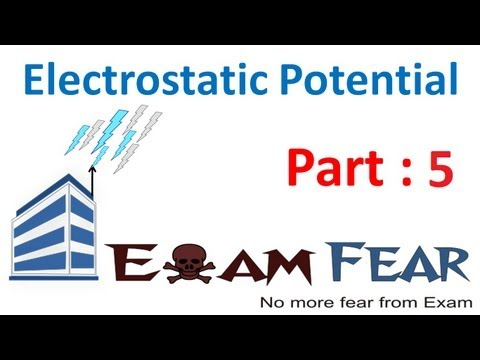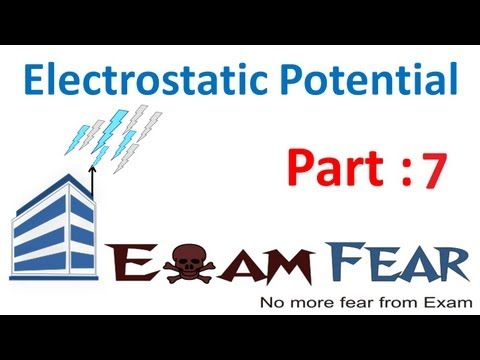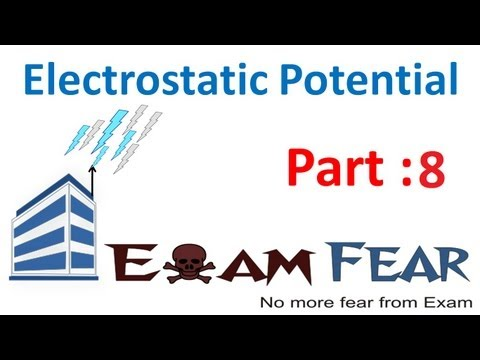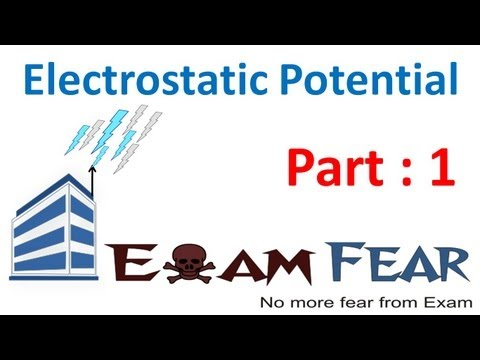### Related tests